Ch 1. Particle General Motion Multimedia Engineering Dynamics Position,Vel & Accel. Accel. varyw/ Time Accel. Constant Rect. Coordinates Norm/Tang. Coordinates Polar Coordinates RelativeMotion
 Chapter - Particle - 1. General Motion 2. Force & Accel. 3. Energy 4. Momentum - Rigid Body - 5. General Motion 6. Force & Accel. 7. Energy 8. Momentum 9. 3-D Motion 10. Vibrations Appendix Basic Math Units Basic Equations Sections Search eBooks Dynamics Fluids Math Mechanics Statics Thermodynamics Author(s): Kurt Gramoll ©Kurt GramollDYNAMICS - THEORY

In the Curvilinear Motion: Rectilinear Coordinates section, it was shown that velocity is always tangent to the path of motion, and acceleration is generally not.

If the component of acceleration along the path of motion is known, motion in terms of normal and tangential components can be analyzed.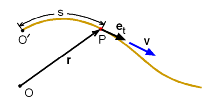Motion of a Particle in a Plane
Plane Motion of a Particle

Consider a point P moving along a curved path.

The position vector r specifies the position of P with respect to the reference point O, and s measures the position of P along the path relative to the reference point O'. The unit tangent vector, et, is tangent to the path at point P.

Velocity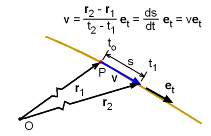Velocity of a Particle in a Plane

The speed of the point along the path is found by taking the derivative of the position,

v = ds/dt

Since the velocity is tangent to the path, it can be expressed in terms of et,

 v = ds/dt et = v et

Acceleration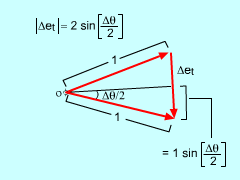Relationship Between Normal
and Tangent Direction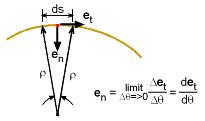Relationship between et and en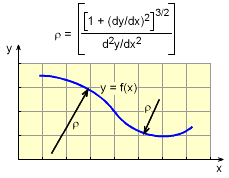Similar to rectilinear coordinates, acceleration is obtained by differentiating the velocity (two parts) as

a = dv/dt = dv/dt et + v det/dt

Since the acceleration is not, in general, tangent to the path, it is useful to express it in terms of components that are normal and tangent to the path. To do so, the time derivative of the unit tangent vector, et, will be found.

Let et(t) be the unit tangent vector at time t, and et(t + Δt) be the unit tangent vector at time t + Δt. If et(t) and et(t + Δt) are drawn from the same origin, they form two radii of length 1 on the unit circle. The magnitude of Δet is given by the equation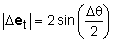Now, consider the vector Δ>et/Δθ. As Δθ approaches 0, Δet/Δθ becomes tangent to the unit circle (perpendicular to the path of P). The magnitude of Δet/Δθ< approaches 1.

Next, define the unit normal vector as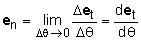The chain rule can be used with the time derivative of the unit tangent vector to give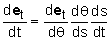But ds/dt = v, det/dθ = en,  and dθ/ds = 1/ρ (where ρ is the radius of curvature). After substituting, the time derivative of the unit tangent vector becomes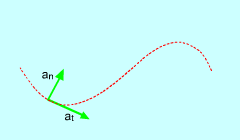Normal and Tangential Acceleration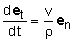The final equation for the acceleration in terms of both normal and tangential components is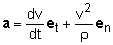Practice Homework and Test problems now available in the 'Eng Dynamics' mobile app
Includes over 400 problems with complete detailed solutions.
Available now at the Google Play Store and Apple App Store.